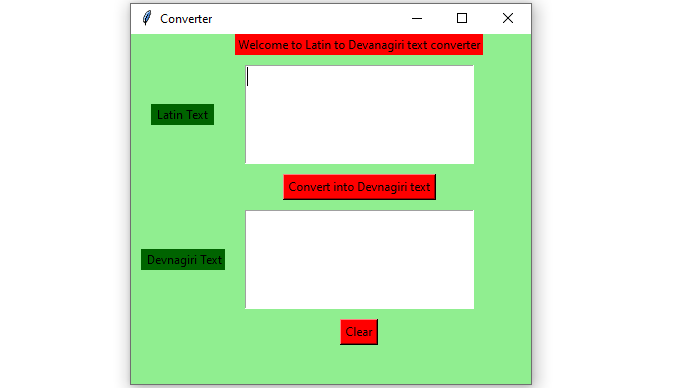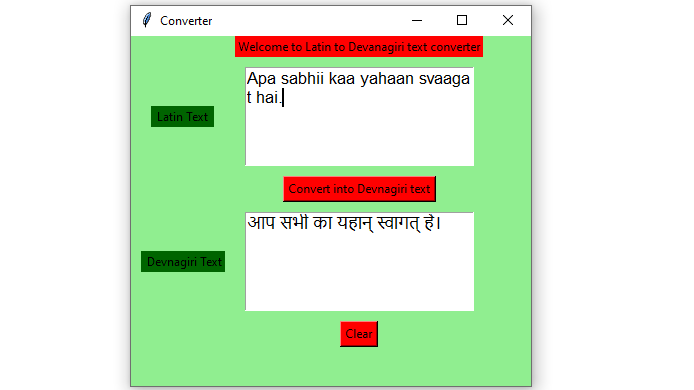Related Articles
Python – English (Latin) to Hindi (Devanagiri) text convertor GUI using Tkinter
• Last Updated : 03 Jul, 2020

Python offers multiple options for developing a GUI (Graphical User Interface). Out of all the GUI methods, tkinter is the most commonly used method. It is a standard Python interface to the Tk GUI toolkit shipped with Python. Python with tkinter outputs the fastest and easiest way to create GUI applications. Now, it’s up to the imagination or necessity of a developer, what he/she wants to develop using this toolkit.

To create a tkinter :

• Importing the module – tkinter
• Create the main window (container)
• Add any number of widgets to the main window.
• Apply the event Trigger on the widgets.

Let’s create a GUI based English(Latin) to Hindi(Devanagari) text-convertor application that can convert text input given by user into Devanagari text.

Below is the implementation :

 `# import sanscript class from the indic_transliteration module``from` `indic_transliteration ``import` `sanscript`` ` `# import transliterate method from sanscript``# class of the indic_transliteration module``from` `indic_transliteration.sanscript ``import` `transliterate`` ` `# import all functions from the tkinter  ``from` `tkinter ``import` `*`` ` `# Function to clear both the text areas``def` `clearAll() :``    ``# whole content of text area  is deleted ``    ``text1_field.delete(``1.0``, END)``    ``text2_field.delete(``1.0``, END)`` ` `# Function to convert into Devanagari text ``def` `convert() :`` ` `    ``# get a whole input content from text box``    ``# ignoring \n from the text box content``    ``input_text ``=` `text1_field.get(``"1.0"``, ``"end"``)[:``-``1``]`` ` `    ``# converted into the given devanagari``    ``# transliterated text``    ``output_text ``=` `transliterate(input_text, sanscript.ITRANS, ``                                            ``sanscript.DEVANAGARI)``   ` `    ``text2_field.insert(``'end -1 chars'``, output_text)`` ` ` ` `# Driver code ``if` `__name__ ``=``=` `"__main__"` `: `` ` `    ``# Create a GUI window ``    ``root ``=` `Tk()`` ` `    ``# Set the background colour of GUI window  ``    ``root.configure(background ``=` `'light green'``)  ``     ` `    ``# Set the configuration of GUI window (WidthxHeight) ``    ``root.geometry(``"400x350"``)  `` ` `    ``# set the name of tkinter GUI window  ``    ``root.title(``"Converter"``)``     ` `    ``# Create Welcome to Latin to Devanagiri text converter``    ``headlabel ``=` `Label(root, text ``=` `'Welcome to Latin to Devanagiri text converter'``,  ``                      ``fg ``=` `'black'``, bg ``=` `"red"``)  ``   ` `    ``# Create a " Latin Text " label  ``    ``label1 ``=` `Label(root, text ``=` `" Latin Text "``, ``                 ``fg ``=` `'black'``, bg ``=` `'dark green'``) ``       ` `    ``# Create a " Devnagiri Text " label  ``    ``label2 ``=` `Label(root, text ``=` `" Devnagiri Text"``,  ``                   ``fg ``=` `'black'``, bg ``=` `'dark green'``)  ``     ` `   ` `    ``# grid method is used for placing  ``    ``# the widgets at respective positions  ``    ``# in table like structure .   ``    ``headlabel.grid(row ``=` `0``, column ``=` `1``)`` ` `    ``# padx keyword argument used to set paading along x-axis .``    ``# pady keyword argument used to set paading along y-axis . ``    ``label1.grid(row ``=` `1``, column ``=` `0``, padx ``=` `10``, pady ``=` `10``)  ``    ``label2.grid(row ``=` `3``, column ``=` `0``, padx ``=` `10``, pady ``=` `10``) `` ` `       ` `    ``# Create a text area box  ``    ``# for filling or typing the information.  ``    ``text1_field ``=` `Text(root, height ``=` `5``, width ``=` `25``, font ``=` `"lucida 13"``) ``    ``text2_field ``=` `Text(root, height ``=` `5``, width ``=` `25``, font ``=` `"lucida 13"``)``        ` `    ``# padx keyword argument used to set paading along x-axis .``    ``# pady keyword argument used to set paading along y-axis .  ``    ``text1_field.grid(row ``=` `1``, column ``=` `1``, padx ``=` `10``, pady ``=` `10``)  ``    ``text2_field.grid(row ``=` `3``, column ``=` `1``, padx ``=` `10``, pady ``=` `10``) `` ` `       ` `    ``# Create a Convert Button and attached  ``    ``# with convert function  ``    ``button1 ``=` `Button(root, text ``=` `"Convert into Devnagiri text"``, ``                     ``bg ``=` `"red"``, fg ``=` `"black"``, command ``=` `convert) ``       ` `    ``button1.grid(row ``=` `2``, column ``=` `1``) ``   ` `    ``# Create a Clear Button and attached  ``    ``# with clearAll function  ``    ``button2 ``=` `Button(root, text ``=` `"Clear"``, bg ``=` `"red"``,  ``                     ``fg ``=` `"black"``, command ``=` `clearAll)``     ` `    ``button2.grid(row ``=` `4``, column ``=` `1``) ``     ` `    ``# Start the GUI  ``    ``root.mainloop()  `

Output :Attention geek! Strengthen your foundations with the Python Programming Foundation Course and learn the basics.

To begin with, your interview preparations Enhance your Data Structures concepts with the Python DS Course. And to begin with your Machine Learning Journey, join the Machine Learning – Basic Level Course

My Personal Notes arrow_drop_up